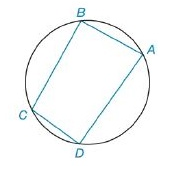Chapter 8.2, Problem 11E### Elementary Geometry for College St...

6th Edition
Daniel C. Alexander + 1 other
ISBN: 9781285195698

#### Solutions

Chapter
Section### Elementary Geometry for College St...

6th Edition
Daniel C. Alexander + 1 other
ISBN: 9781285195698
Textbook Problem
1 views

# For Exercises 11 and 12, use Brahmagupta’s Formula.For cyclic quadrilateral A B C D , find the area if A B   =   39 mm, B C   =   52 mm, C D   =   25 mm, and D A   =   60 mm.To determine

To Find:

The area of a cyclic quadrilateral using Brahmagupta’s Formula.

Explanation

Formula Used:

For a cyclic quadrilateral with sides of lengths a,b,c and d, the area A is given by

A=(s-a)(s-b)(s-c)(s-d),

where s=12(a+b+c+d).

Calculation:

Consider, AB=a=39 mm, BC=b=52 mm CD=c=25 mm and DA=d=60 mm.

s=1239+52+25+60=88

### Still sussing out bartleby?

Check out a sample textbook solution.

See a sample solution

#### The Solution to Your Study Problems

Bartleby provides explanations to thousands of textbook problems written by our experts, many with advanced degrees!

Get Started

#### Find the domain of the function. a. f(x) = 9x b. f(x) = x+32x2x3

Applied Calculus for the Managerial, Life, and Social Sciences: A Brief Approach

#### Find for .

Study Guide for Stewart's Multivariable Calculus, 8th

#### Which is the best graph of r = 1 − sin θ for 0 ≤ θ ≤ π?

Study Guide for Stewart's Single Variable Calculus: Early Transcendentals, 8th